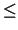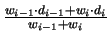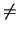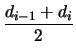Next: C. The Gummel-Poon BJT Up: MINIMOS-NT Previous: A. Carrier Interface Models

# B. The Akima Interpolation

The Akima interpolation  is a continuously differentiable sub-spline interpolation. It is built from piecewise third order polynomials. Only data from the next neighbor points is used to determine the coefficients of the interpolation polynomial. There is no need to solve large equation systems and therefore this interpolation method is computationally very efficient. Because no functional form for the whole curve is assumed and only a small number of points is taken into account this method does not lead to unnatural wiggles in the resulting curve. The monotonicity of the specified data points is not necessarily retained by the resulting interpolation function. By additional constraints on the estimated derivatives a monotonicity preserving interpolation function can be constructed .

For a set of data points

 si = s(xi),        1ik, (B1)

the interpolation function is defined as

 s(x) = a0 + a1 . (x - xi) + a2 . (x - xi)2 + a3 . (x - xi)3,        xixxi + 1. (B2)

To determine the coefficients a0, a1, a2, and a3 of the interpolation polynomial for each interval [xi, xi + 1] the function values si and si + 1, and the first derivatives si' and si + 1' at the end points of the interval are used.

The first derivative si' of the interpolation function at xi is estimated from the data for this point and the next two points on each side of xi. Using the ratios

 dj =,        j = i - 2, i - 1, i, i + 1, (B3)

and the weighting coefficients

 wi - 1 = | di + 1 - di|, (B4) wi = | di - 1 - di - 2|, (B5)

the estimated derivative si' is defined as

 si' =. (B6)

Several special cases for si' have to be considered.

 si' = di - 1 di - 2 = di - 1, didi + 1 (B7) si' = di di = di + 1, di - 2di - 1 (B8) si' = di - 1 = di di - 1 = di (B9) si' =di - 2 = di - 1di = di + 1 (B10)

To be able to use (B.6) for calculating the derivatives s1', s2', sk - 1', and sk' additional ratios d-1, d0, dk, and dk + 1 have to be estimated.

 d-1 = 2 . d0 - d1 (B11) d0 = 2 . d1 - d2 (B12) dk = 2 . dk - 1 - dk - 2 (B13) dk + 1 = 2 . dk - dk - 1 (B14)

The order of the interpolation function reduces to 2 for these intervals.

Similar algorithms can be used for the interpolation of two-dimensional data on rectangular grids  and on unstructured grids by bicubic and cubic polynomials, respectively.Next: C. The Gummel-Poon BJT Up: MINIMOS-NT Previous: A. Carrier Interface Models
Martin Rottinger
1999-05-31